Next: ET_objective.m Up: Subsidiary calculation functions Previous: desilets2006sp.m   Contents

## dunai2001sp.m

Syntax:

scalingfactor = dunai2001sp(h,Rc)


Calculates the geographic scaling factor for cosmogenic-nuclide production for particular cutoff rigidity and atmoshperic pressure according to the scheme in:

Dunai T.J., 2001. Influence of secular variation of the geomagnetic field on production rates of in situ produced cosmogenic nuclides. Earth and Planetary Science Letters, v. 193, pp. 197-212.

The input arguments are h, atmospheric pressure (hPa) and Rc, cutoff rigidity (GV). Accepts vector arguments.

This function:

Converts atmospheric pressure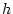(hPa) to atmospheric depth between sea level and the site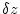(g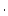cm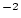) by: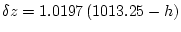(33)

Calculates the sea level scaling factor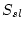according to Equation (3) of the source paper: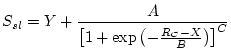(34)

where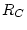is the cutoff rigidity (GV) and the other constants in the equation are: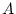0.5221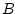-1.7211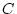0.3345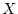4.2822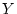0.4952

Calculates the atmospheric attenuation length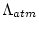according to Equation (4) of the source paper: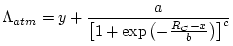(35)

whereis the cutoff rigidity (GV) and the other constants in the equation are: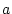17.183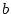2.06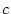5.9164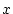2.2964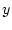130.11

Finally, calculates the scaling factor at the site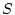according to Equation (5) of the source paper: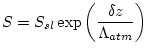(36)

Accepts either scalars or vectors of equal sizes for all the input arguments. Returns either a scalar or a vector of the appropriate size.Next: ET_objective.m Up: Subsidiary calculation functions Previous: desilets2006sp.m   Contents
2007-11-13